Question Pool Refraction of Light

# Refraction of Light - SAMAGRA Question Pool & Answers | Class 10 English Medium

Kerala Syllabus SAMAGRA SCERT SAMAGRA Question Pool for Class 10 English Medium Physics Refraction of LightQn 1.

The critical angle of glass is 42? .

1. What is meant by Critical Angle ?
2. When the angle of incidence in glass is 42? what will be the angle of refraction ?
3. Name the Phenomenon occur when the angle of incidence is 40?, Define this phenomenon.
4. Name the Phenomenon occur when the angle of incidence is 45?, Define this phenomenon.
1. When a ray of light passes from a medium of greater optical
density to that of lower optical density, the angle of incidence
at which the angle of

Get Free Study Materials + 1 Week Free Trial of BrainsPrep Class 10 English Medium Tuition

Qn 2.

Pick out the wrong statements from the following and rewrite them after correction.

1. Refraction is due to the difference in the optical density of different media.
2. Velocity of light is greater in the media with higher optical density.
3. Optical density of glass is less than that of water.
4. Velocity of light in vacuum is 3 x 108 m/s.

wrong statements b,c

Get Free Study Materials + 1 Week Free Trial of BrainsPrep Class 10 English Medium Tuition

Qn 3.

Find the magnification of the image formed when the object is placed at 2F of a convex lens.

(Greater than 1, 1 ,Less than 1, 0)

Magnification=1 (one)

Get Free Study Materials + 1 Week Free Trial of BrainsPrep Class 10 English Medium Tuition

Qn 4.

A person use a spectacle of power of lens -1.25 D.

1. What type of lens is this?
2. What is mean by power of a lens?
3. Find the focal length of the lens ?

Get Free Study Materials + 1 Week Free Trial of BrainsPrep Class 10 English Medium Tuition

Qn 5.

Analyse the ray diagram. OB indicates an object placed in front of the convex lens.

imAGE

1. Complete the ray diagram using given incident rays and find the position of the image formed.
2. What will be the position of image when the object is placed at 2F.
3. Where should be the object placed to get a virtual image ?

Get Free Study Materials + 1 Week Free Trial of BrainsPrep Class 10 English Medium Tuition

Qn 6.

The diagram below is of an object OB placed in front of a lens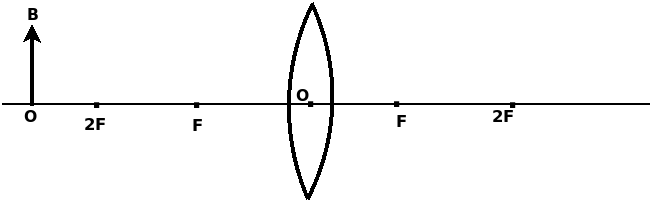1. Which type of lens is used here ?
2. Complete the ray diagram and find the position of the image.
3. Write any two characteristics of the image.

Get Free Study Materials + 1 Week Free Trial of BrainsPrep Class 10 English Medium Tuition

Qn 7.

Complete the diagram and label the principal focus of the concave lens.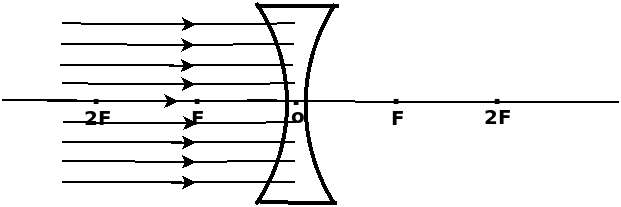Get Free Study Materials + 1 Week Free Trial of BrainsPrep Class 10 English Medium Tuition

Qn 8.

An image with twice the size of the object is produced on a screen using a convex lens of focal length 15cm.

1. What is the Object distance from lens ?
2. What is the Image distance from lens ?

Get Free Study Materials + 1 Week Free Trial of BrainsPrep Class 10 English Medium Tuition

Qn 9.

Statements related to the images formed by lenses are given below. Tabulate them into those related to real images and those related to virtual images.

1. Inverted
2. cannot be captured on screen
3. can be captured on a screen
4. image formed when actual intersection of light rays occur
5. erect
6. magnification will be negative

Real images- a,c,d,f

Get Free Study Materials + 1 Week Free Trial of BrainsPrep Class 10 English Medium Tuition

Qn 10.

The image of a lit candle is produced on a screen using convex lens. Find the position of the object in each of the following conditions.

1. Obtains an image of size equal to the object .
2. Obtains  an image smaller than object.
3. Obtains an real image bigger than the object.
1. 2F Or C
2. Beyond 2F Or Beyond C
3. In between 2F and

Get Free Study Materials + 1 Week Free Trial of BrainsPrep Class 10 English Medium Tuition

Qn 11.

The terms given are related to lens. Using these fill up the following statements.

(Focal length, Pricipal axis, Optic centre, Centre of curvature, Radius of curvature.)

1. ...............is the centre of the lens.
2. The distance between the optic centre and the principal focus is............
3. The centre of a sphere of which  lens is a part is known as..............................
4. The imaginary line joining the two centres of curvature of the lens and passing through the optic centre is ......................................................

1. Pole
2. Focal length
3. Centre of

Get Free Study Materials + 1 Week Free Trial of BrainsPrep Class 10 English Medium Tuition

Qn 12.

The light lays falling parallel to the principal axis of a convex lens is shown in the diagram. Complete the diagram & label the principal focus of the lens.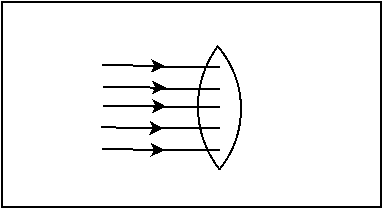Get Free Study Materials + 1 Week Free Trial of BrainsPrep Class 10 English Medium Tuition

Qn 13.

Light ray entering glass from air at an angle is shown in the diagram. Which among the following is the correct one.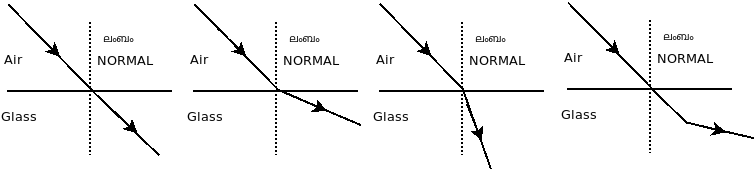i                           ii                          iii

Figure iii, It bents towards the normal

Get Free Study Materials + 1 Week Free Trial of BrainsPrep Class 10 English Medium Tuition

Qn 14.

The diagrams show light ray entering glass slab from air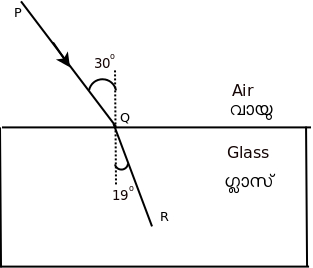Analyse these diagrams and answer the following questions.

1. Which is the incident ray ?
2. Which is the refracted ray ?
3. Which is the angle of incidence ?
4. Which is the angle of refraction ?
5. What happens to the light ray when it enter the glass from air at an angle.
6. Which is this phenomenon?
1. PQ
2. QR
3. 300
4. 190
5. It bend towards the normal (refraction takes

Get Free Study Materials + 1 Week Free Trial of BrainsPrep Class 10 English Medium Tuition

Qn 15.

Observe the figure. XY is the surface of separation of the media A and B. Light ray enters B from A

..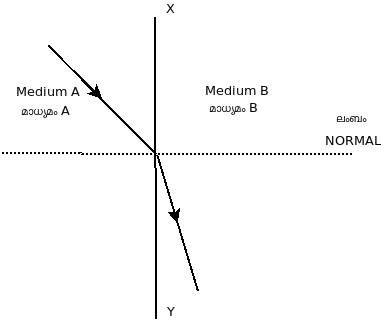1. When the light ray enter B from A.                                                                       (no deviation, move towards the normal, moves away from the normal)
2. Among media A& B. Which has more optical density?
3. In which medium does the light travels with more velocity ?
1. It bent towards the normal
2. Medium B

Get Free Study Materials + 1 Week Free Trial of BrainsPrep Class 10 English Medium Tuition

Qn 16.

Find the relation and complete the word pair                                                                Focal length : metre                                                                                                      Power of lens : ............

Power of lens : Dioptre

Get Free Study Materials + 1 Week Free Trial of BrainsPrep Class 10 English Medium Tuition

Qn 17.

Find the power of a convex lens with focal length 10cm

Get Free Study Materials + 1 Week Free Trial of BrainsPrep Class 10 English Medium Tuition

Qn 18.

Object AB is placed in front of a concave lens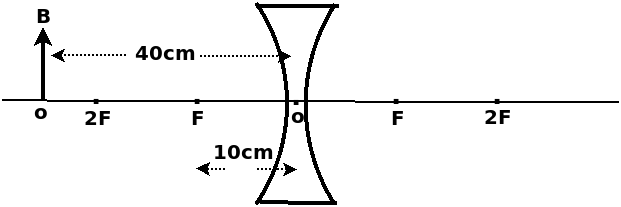1. What is the focal length of the lens in New Cartesian sign convention.
2. Calculate the image distance

Get Free Study Materials + 1 Week Free Trial of BrainsPrep Class 10 English Medium Tuition

Qn 19.

An object is placed 20 cm from the convex lens, virtual and erect images formed 100 cm from the lens.

1. Find out the focal length of the lens ?
2. What is the power of the lens?

Get Free Study Materials + 1 Week Free Trial of BrainsPrep Class 10 English Medium Tuition

Qn 20.

The diagram shows the image formation by a convex lens.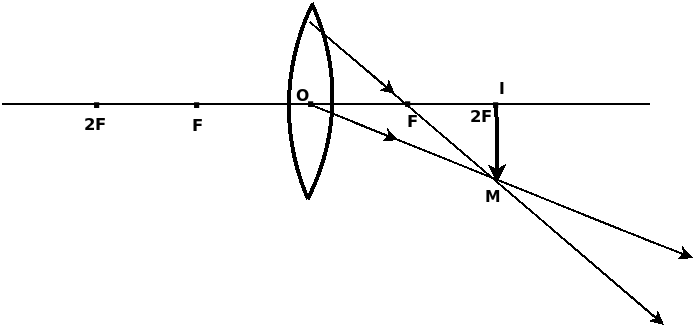1. Find the position of the image by completing the ray diagram.

Get Free Study Materials + 1 Week Free Trial of BrainsPrep Class 10 English Medium Tuition

Qn 21.

The diagram shows the image formation by a convex lens.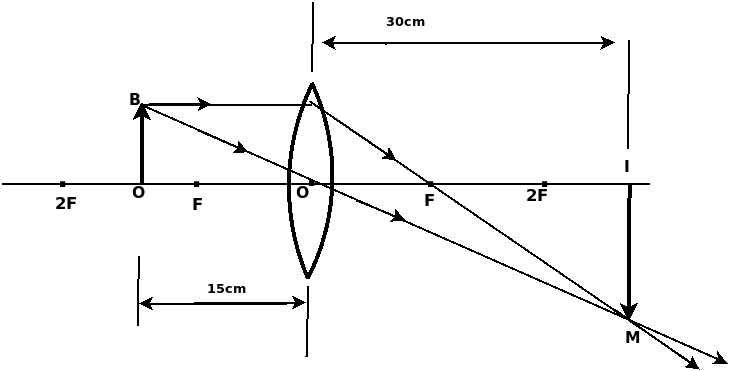1. Find out the focal length using New Cartesian method.
2. Find its magnification using ray diagram?

Get Free Study Materials + 1 Week Free Trial of BrainsPrep Class 10 English Medium Tuition

Qn 22.

Express the following measurements in New Cartesian Sign Convention. IM is the image of object OB.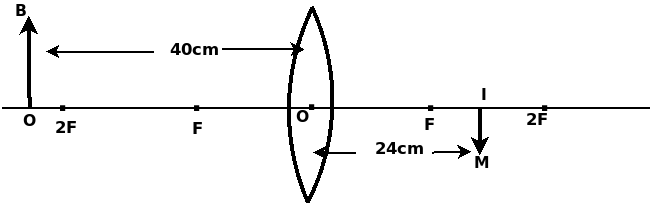1. Object distance (u) = ________
2. Image distance (v) = ________
3. Height of the Object (OB) = ________
4. Height of the Image (IM) = ________
1. u = - 40 cm
2. v = +24 cm
3. OB = +2 cm
4. IM = - 1

Get Free Study Materials + 1 Week Free Trial of BrainsPrep Class 10 English Medium Tuition

Qn 23.

Method of measuring distances according to New Cartesian Sign Convention is given below. Choose the correct statements from these.

1. All the distances are measured form F.
2. The distances measured in the direction of incident ray are positive.
3. It is assumed that the incident rays travels from right to left.
4. X axis is considered as the principal axis.

b. All distances measured along the direction of incident light is positive.

Get Free Study Materials + 1 Week Free Trial of BrainsPrep Class 10 English Medium Tuition

Qn 24.

Observe the figure carefully and an object ‘OB’ is placed in front of the concave lens

.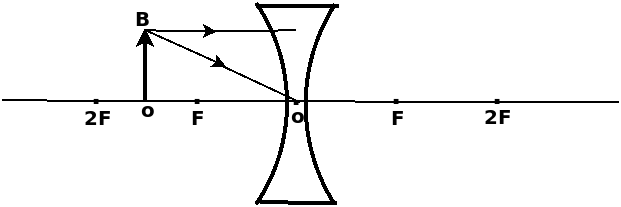1. Find the position of the image by completing the diagram?
2. Can this image be screened? why?
3. If the position of the object is in 2 F, where is the position of the image?

Get Free Study Materials + 1 Week Free Trial of BrainsPrep Class 10 English Medium Tuition

Qn 25.

An object is placed in front of the lens is given below.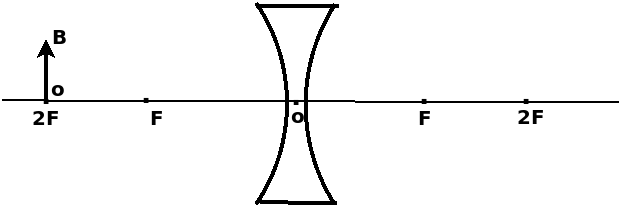1. Whether the principal focus of this lens is real or virtual .
2. Find out the position of the image by completing the figure.
3. Write any two nature of the image.

Get Free Study Materials + 1 Week Free Trial of BrainsPrep Class 10 English Medium Tuition

Qn 26.

Observe the figure given below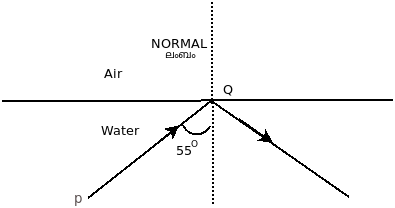1. Why the ray PQ reflected this way?
2. Name the phenomenon?
3. What will happen to the ray of light when angle of incidence is 300 at Q
1. Angle of incidence is greater than that of critical angle.
2. Total internal

Get Free Study Materials + 1 Week Free Trial of BrainsPrep Class 10 English Medium Tuition

Qn 27.

Obseve the table and answer the questions given below

.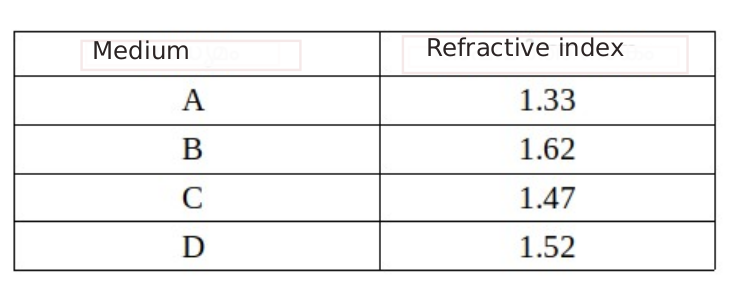1. In which medium the speed of light is maximum?
2. Which of the following is correct based on the speed of light in the media?          1) A>B>D>C    2) A>C>B>D    3) A>C>D>B     4) A<C<D<B

c. Find out the speed of light in medium B?(speed of light in vaccum = 3x108m/s )

1. Mediuum A
2. 3) A >C> D>B
3. n= c/v

Get Free Study Materials + 1 Week Free Trial of BrainsPrep Class 10 English Medium Tuition

Qn 28.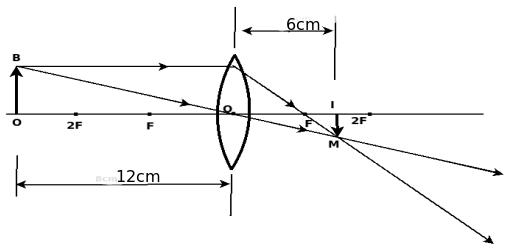1. Find u and v in terms of new cartesian sign convention?
2. Is the image real?
3. Find out the focus of the lens from  the diagram.Justify the answer by using lens formula

Get Free Study Materials + 1 Week Free Trial of BrainsPrep Class 10 English Medium Tuition